# ECMAScript Loops Tutorial

## 1- Overview of the loops in ECMAScript

In ECMAScript, statements are executed sequentially from top to bottom. However, when you want to execute a sequence of statements multiple times, you can use loop.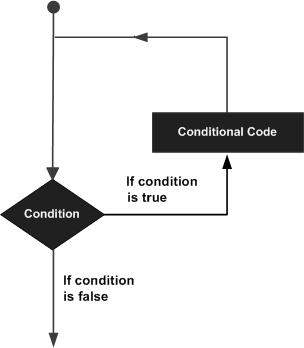ECMAScript provides you with 3 types of loops:
1. while loop
2. for loop
3. do .. while loop
Statements can be used within a loop:
1. continue
2. break
Control Statement Description

break

Terminates the loop statement.

continue

Causes the loop to skip the remainder of its body and immediately retest its condition prior to reiterating.

## 2- while loop

The syntax of a  while loop:
```while (condition)  {
// Do something here
// ....
}
```
Example:
while-loop-example.js
```console.log("While loop example");
// Declare a variable, and assign value of 2.
let x = 2;

// Condition is x < 10
// If x < 10 is true then run block
while (x < 10) {

console.log("Value of x = ", x);

x = x + 3;
}

// This statment is out of while block.
console.log("Finish");
```
Running the example: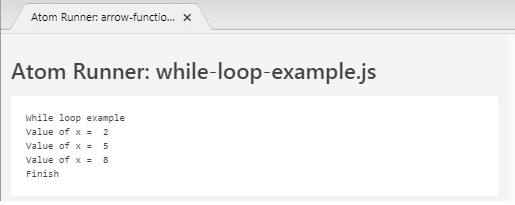## 3- for loop

The standard   for loop has the syntax as follows:
```for(InitialValues; condition; updateValues )
{
// Statements
}
```
• InitialValues: Initialize  values for the related variables in the loop.
• condition: Defines the conditions for executing command blocks.
• updateValues: Update new values for variables
for-loop-example.js
```
console.log("For loop example");

for( let i = 0; i < 10; i= i + 3)  {

console.log("i= "+ i);
}
```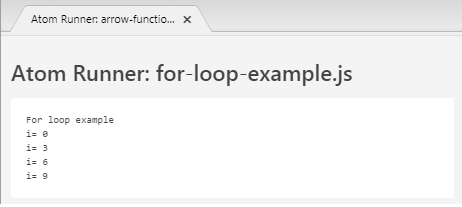for-loop-example2.js
```console.log("For loop example");

for(let i = 0, j = 0; i + j < 10; i = i+1, j = j+2)  {

console.log("i = " + i +", j = " + j);
}
```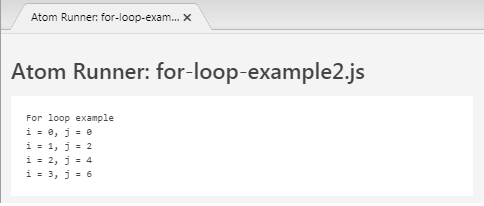Using the for loop can help you traverse on the elements of the array.
for-loop-example3.js
```console.log("For loop example");

// Array
let names =["Tom","Jerry", "Donald"];

for (let i = 0; i < names.length; i++ ) {

console.log("Name = ", names[i]);
}

console.log("End of example");
```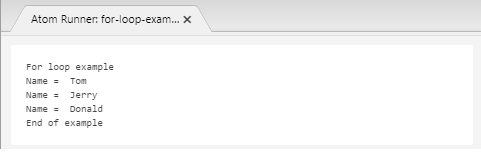## 4- For .. in loop

The  for..in loop helps you iterate over the  properties of an object.
```
for (prop in object) {

// Do something

}

```
Example:
for-in-loop-example.js
```
// An object has 4 properties (name, age, gender,greeing)
var myObject = {
name : "John",
age: 25,
gender: "Male",
greeting : function() {
return "Hello";
}
};

for(myProp in myObject)  {

console.log(myProp);
}
```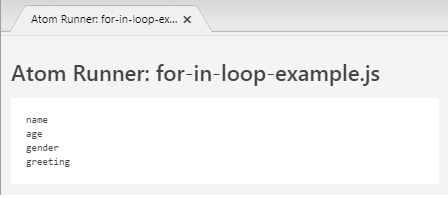## 5- For .. of loop

The for..of loop helps you iterate over a Collection, for example,  Map, Set.
Example:
for-of-example.js
```// Create a Set from an Array
var fruits = new Set( ["Apple","Banana","Papaya"] );

for(let fruit of fruits)  {

console.log(fruit);

}
```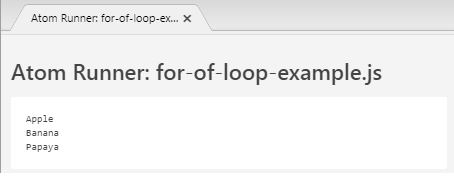for-of-example2.js
```// Create a Map object.
var myContacts = new Map();

myContacts.set("0100-1111", "Tom");
myContacts.set("0100-5555", "Jerry");
myContacts.set("0100-2222", "Donald");

for( let arr of myContacts)  {
console.log(arr);
console.log(" - Phone: " + arr);
console.log(" - Name: " + arr);
}

```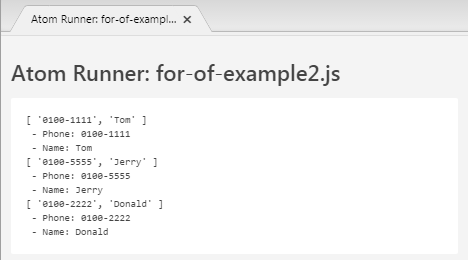## 6- do...while loop

The do-while loop is used to execute a section of program many times.The characteristics of the do-while is that the block of statement is always executed at least once. After each iteration, the program will check the condition, if the condition is still correct, the statement block will be executed again.
```do {
// Do something
}
while (expression);
```
Example:
do-while-loop-example.js
```let value = 3;

do {
console.log("Value = " + value);

value = value + 3;

} while (value < 10);
```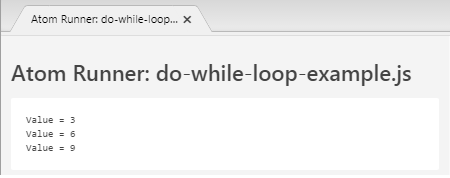## 7- Use the break statement in the loop

break is a statement that may be located in a loop. This statement ends the loop unconditionally.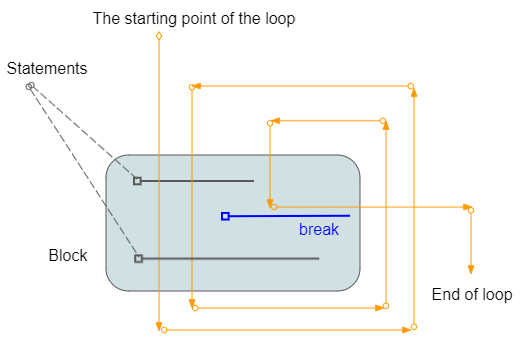loop-break-example.js
```console.log("Break example");

// Declare a variable and assign value of 2.
let x = 2;

while (x < 15) {
console.log("----------------------\n");
console.log("x = ", x);

// If x = 5 then exit the loop.
if (x == 5) {
break;
}

// Increase value of x by 1
x = x + 1;
console.log("x after + 1 = ", x);
}

console.log("End of example");
```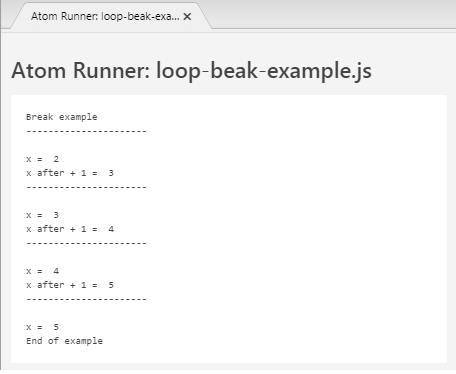## 8- Use the continue statement in the loop

continue is a statement which may be located in a loop. When caught the continue statement, the program will ignore the command lines in block, below of  continue and start of a new loop.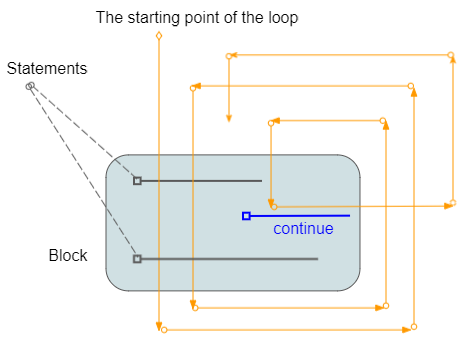loop-continue-example.js
```console.log("Continue example");

// Declare a variable and assign value of 2
x = 2

while (x < 7) {
console.log("----------------------\n")
console.log("x = ", x)

// % is used for calculating remainder
// If x is even, then ignore the command line below of continue
// and start new iteration.
if (x % 2 == 0) {
// Increase x by 1.
x = x + 1;
continue;
}
else {
// Increase x by 1.
x = x + 1

console.log("x after + 1 =", x)
}
}

console.log("End of example");
```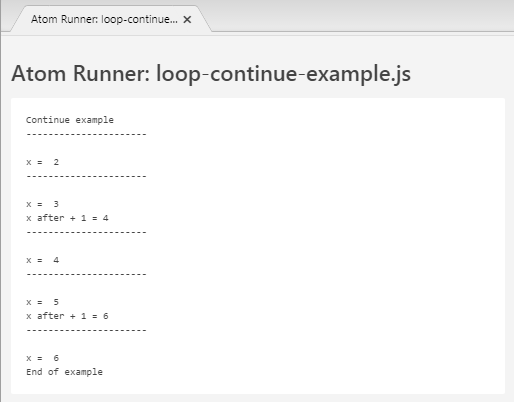## 9- Labelled Loop

The ECMAScript allows you to stick a label to a loop. It is like you name a loop, which is useful when you use multiple nested loops in a program.
• You can use break labelX statement; to break a loop is attached labelX.
• You can use continue labelX statement; to continue a loop is attached​​​​​​​ labelX.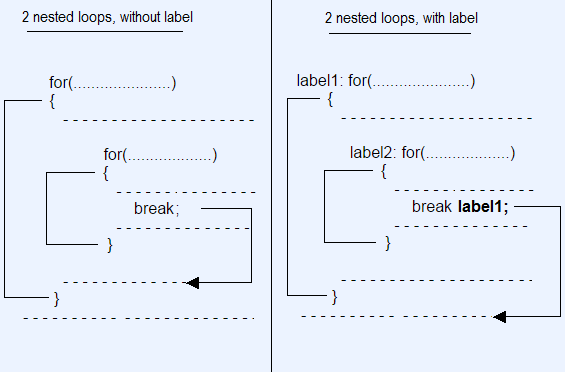Syntax:
```// for loop with Label.
label1: for( ... )  {

}

// while loop with Label.
label2: while ( ... )  {

}

// do-while loop with Label.
label3: do  {

} while ( ... );

```
Example of using nested loops, labelled and labelled break statement.
labelled-loop-break-example.js
```console.log("Labelled Loop Break example");

let i = 0;

label1: while (i < 5) {

console.log("----------------------\n");
console.log("i = " + i);
i++;

label2: for (let j = 0; j < 3; j++) {

console.log("  --> " + j);
if (j > 0) {
// Exit the loop with label1.
break label1;
}
}

}

console.log("Done!");
```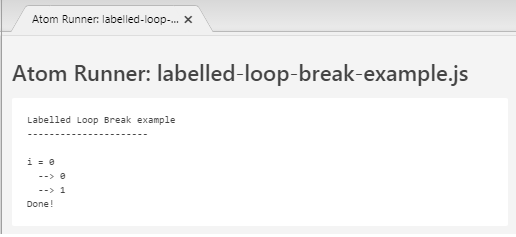Example of using nested labelled loops and labelled continue statement.
labelled-loop-continue-example.js
```let i = 0;

label1: while (i < 5) {
console.log("outer i= " + i);
i++;

label2: for (let j = 0; j < 3; j++) {
if (j > 0) {

continue label2;
}
if (i > 1) {

continue label1;
}
console.log("inner i= " + i + ", j= " + j);
}

}
```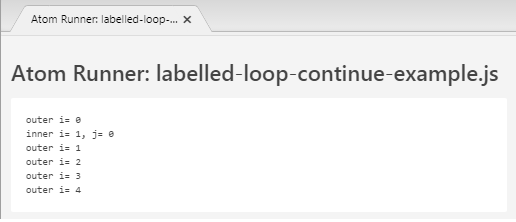#### View more Tutorials:

Maybe you are interested

These are online courses outside the o7planning website that we introduced, which may include free or discounted courses.

•Essentials of JavaScript Practice Coding Exercises Tips
•Getting really good at JavaScript and TypeScript
•Learn JavaScript From Scratch:Become Top Rated Web Developer
•VueJS V1 Introduction to VueJS JavaScript Framework
•JavaScript Dynamic Quiz Application from Scratch JSON AJAX
•Learn JavaScript Fundamentals
•HTML5, CSS3 And JavaScript Fundamentals 2016
•2D Game Development With HTML5 Canvas, JS - Tic Tac Toe Game
•Build a Real Time Chat App with JavaScript and Firebase
•Starting with JavaScript Unit Testing
•JavaScript 101 Gain insights how to code with JavaScript
•*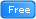* Introductory To JavaScript - Learn The Basics of JavaScript
•Javascript for Beginners
•Master ExpressJS to Build Web Apps with NodeJS&JavaScript
•Learn Javascript from Scratch - JS Tutorial for beginners